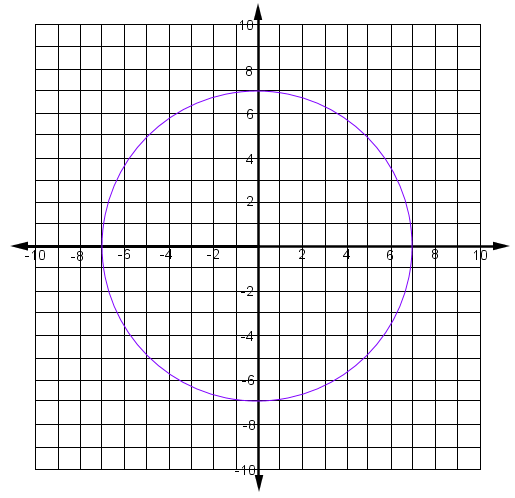Home > CCG > Chapter 12 > Lesson 12.2.2 > Problem12-74

12-74.

Find the area of the graph of the solution region of $x^2 + y^2 < 49$. Homework Help ✎

What is the radius?
What is the formula for the area of a circle?

$49\pi$ un$^2$